近日，哈弗品牌公布了12月销量数据，销量再创新高，达到107,578辆，在逆势四连涨的强势助推下，2018年哈弗累计销量高达766,062辆，继续稳坐中国SUV销冠宝座，并创下了连续9年蝉联中国SUV销量第一的记录，同时更是成功实现全球500万辆销量，成为首个以最快速度达到500万辆的中国SUV品牌。

以上种种耀眼成绩，可谓尽显哈弗SUV领导者的霸主本色，大大提振了市场信心，也为中国汽车市场的发展注入了一剂“强心剂”，持续引领自主品牌不断向上突破。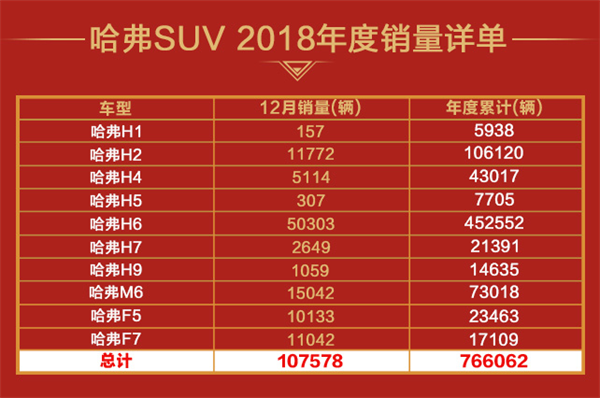回顾过去一年，受宏观经济、中美贸易战等因素的影响，国内乘用车市场增速放缓，在如此激烈的市场竞争中，哈弗品牌却稳如泰山，销量持续稳步提升。一方面源哈弗坚持“聚焦SUV”战略，不断为消费者提供卓越的产品品质及极致的用车体验，更重要的是，在如今年轻化已经成为时代主旋律的背景下，哈弗在2018年以当前市场需求为导向，顺势而为，主动出击，推出一系列多元化营销策略，为品牌注入了新活力的同时，助推哈弗品牌向上进阶。

进军“娱乐圈”，哈弗一鸣惊人，品牌活力迅猛提升

目前，90后年轻一代已成为汽车市场消费的中流砥柱，且随着人们生活消费水平日益提高，“娱乐+消费”的模式逐渐成为一种趋势。在此背景下，如何通过多元化的营销抓住年轻消费者的注意力就显得极为重要。哈弗深谙此道，为了更好的和年轻人打成一片，2018年哈弗积极顺应市场发展趋势，在娱乐营销上全力出击。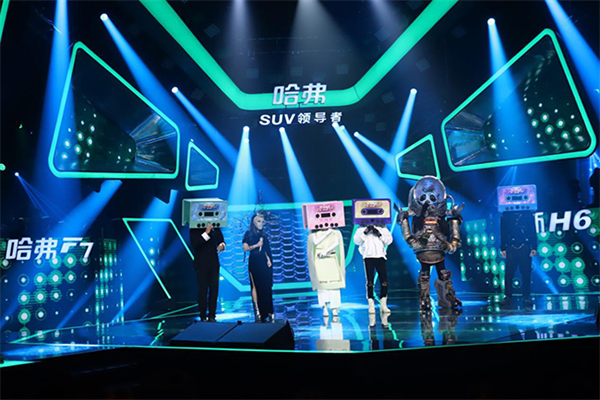2018新年伊始，哈弗品牌牵手湖南卫视2017-2018跨年演唱会开启新年大事件，打响2018娱乐营销第一枪。哈弗此次高调进入“娱乐圈”，以全新的形象走进公众视野，迅速获得市场及消费者的热烈响应，实现了品牌传播最大化。为了进一步加强与年轻消费者的沟通、交流，哈弗品牌将娱乐营销贯彻到底，在2018年还相继冠名了大热IP《蒙面唱将猜猜猜》，赞助了明星家庭真人秀《最美的时光》，通过这一系列的娱乐营销活动，不仅为品牌注入了年轻活力，同时更是将哈弗产品与消费者的情感建立联系，使哈弗品牌的声量大幅提升，为提升品牌影响力和销量均起到了至关重要的作用。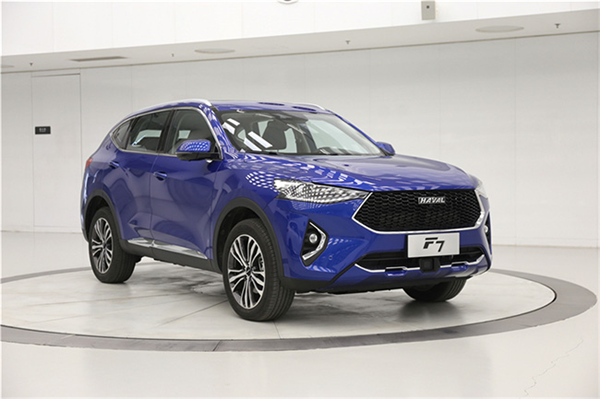随着哈弗不断拉进与年轻消费者间的距离，同时凭借哈弗强大的产品研发实力以及对市场的敏锐洞察，立刻推出了面向年轻消费群体的F系产品，H、F双剑合璧，使哈弗产品矩阵更加丰富，同时更是将年轻化迅速落地，且F系一经推出就获得市场高度认可，在12月，哈弗F5、哈弗F7销量更是双双破万，锋芒毕露，再次向市场展示了哈弗品牌精准的战略眼光。

相得益彰，哈弗借势世界杯，彰显品牌冠军精神

2018年哈弗在娱乐营销方面一鸣惊人的同时，在体育营销上同样大放光彩。对于长期稳坐中国SUV市场冠军宝座的哈弗品牌，冠军精神早已刻进品牌DNA，为了将品牌文化与体育精神相融合，让年轻、活力、激情的品牌形象深入人心，哈弗在2018年一举成为央视世界杯转播顶级合作伙伴，通过与央视平台的深度合作为品牌传播再添重磅砝码。同时，为了多维度挖掘冠军精神内涵，"2018哈弗寻冠之旅"在世界杯期间应运而生，让更多哈弗粉丝在精心设计的活动过程中，领悟哈弗冠军精神的力量。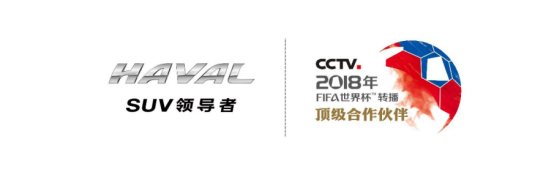拼搏向上，坚持不懈，团队荣誉，这是世界杯所倡导的精神，而哈弗品牌短短十几年，从诞生到如今中国第一且全球销量领先的SUV品牌，哈弗品牌又何尝不是在向消费者诠释这种精神？

哈弗品牌之所以能够如此成功，究其原因，得益于自诞生之日起就始终坚持的“聚焦SUV战略”，以品质为核心驱动，集研发、技术、产业链等优势资源于一体为消费者打造高品质SUV。同时哈弗持续以消费者为中心，致力为用户提供优异的产品和完善的服务，并且积极求变，凭借着雄厚的技术储备，通过对产品的不断优化、完善，全方位保持市场竞争中的王者地位，最终成为了自主品牌的主导力量，强势引领了中国SUV潮流的兴起。

哈弗借势2018年俄罗斯世界杯，为品牌打造了一场体育营销大事件，不仅让哈弗向品牌年轻化转型更进一步，同时更是充分向消费者展示了哈弗品牌的冠军精神。

钜惠促销深入人心，强势助力全球500万销量达成

毫无疑问，2018年哈弗把年轻化元素融入品牌基因，与年轻人同频共振，在娱乐营销与体育营销的合力助推下，将直击广大年轻消费者心底，使哈弗品牌的声量大幅提升。与此同时，为了圆更多消费者的高品质SUV梦，哈弗自9月起推出了“纯自主迎贸易战 哈弗神车降2万”等一系列的钜惠促销活动，大幅让利消费者，更强化了哈弗SUV的竞争力，进一步扩大市场份额。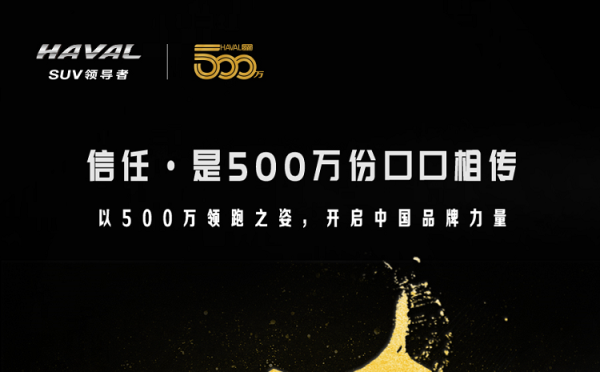在此背景下，哈弗开启了一波独立行情，连续四个月实现销量稳步增长，助力哈弗品牌创下了连续9年蝉联中国SUV销量第一的记录，且在2018年终之际，哈弗品牌更是成功实现全球500万辆销量，成为首个以最快速度达到500万辆的中国SUV品牌。哈弗能在如此激烈残酷的市场竞争中，发起巨额让利活动，并最终取得这一系列傲人成绩。这是哈弗顺应市场以及时代潮流的创新举措，展现了哈弗多年以来对市场的准确预判和把握，充分彰显了哈弗品牌的SUV领导者的王者实力，可谓强者恒强。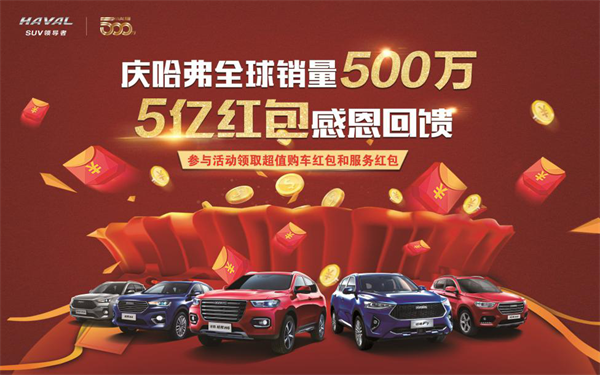为了感恩回馈全球500万用户的信赖与支持，在刚刚结束的500万盛典中，哈弗正式上线了“庆哈弗全球销量500万，5亿红包感恩回馈”活动。即日起至2月28日，哈弗为即将购车的客户提供了1000元、2000元、3000元、4000元、4999元五档价值不等的购车红包，为车主提供包括维保券、精品券、延保/保险券等多种服务红包，总金额高达5亿元。

结语：

哈弗品牌自诞生就坚持“聚焦SUV战略”，致力为消费者打造高品质SUV。在此基础上，哈弗砥砺前行，不断优化完善产品力、产品布局，使哈弗品牌综合竞争力遥遥领先。

但酒香也怕巷子深，尤其在今年市场增速放缓的情况下，如何抢占先机致胜市场，就显得尤为重要。哈弗深谙此道，2018年通过多维度花式营销，先发夺人，主动出击，抢占更多市场份额，夯实市场领先地位的同时，为品牌注入年轻活力，强势助力哈弗品牌2018年屡获佳绩，伴随着哈弗再次夺得中国SUV市场销量冠军以及作为中国首个以最快速度达到500万辆的中国SUV品牌，2018哈弗完美收官。

##### 相关资讯

|分享

•已阅！握爪
•我手滑为你点赞
•128个赞！
•不明觉厉
•阅后既醉
•有钱！任性
•照片太美，我不敢看
•干货！杠杠的
•高大上
•膜拜中！
•请接受我的膝盖
•猫哥/猫妹么么哒
•神吐槽
•我只笑笑不说话
•我想静静
•窒息
##### 资讯相关车系##### 热度排行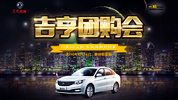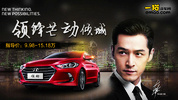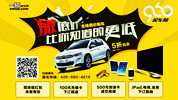• 阿斯顿·马丁
• 奥迪
• 阿尔法罗密欧
• ALPINA
• 爱驰
• AC Schnitzer

• 巴博斯
• 宝骏
• 宝马
• 保时捷
• 北汽制造
• 奔驰
• 奔腾
• 本田
• 比亚迪
• 标致
• 别克
• 宾利
• 布加迪
• 北汽威旺
• 北京
• 北汽绅宝
• 北汽幻速
• 北汽新能源
• 宝沃
• 比速汽车
• 北汽道达
• 北京汽车
• 比德文汽车
• 铂驰
• 北汽昌河

• 昌河
• 长安
• 长城
• 长安商用
• 成功汽车
• 长江EV
• 长安轻车型
• 长安欧尚
• 长安凯程
• 长安跨越
• 车驰汽车

• 大众
• 道奇
• 东风
• 东风风神
• 东风小康
• 东南
• DS
• 东风风行
• 东风风度
• 东风风光
• 电咖
• 大乘汽车
• 大运
• 东风富康

• 法拉利
• 菲亚特
• 丰田
• 福迪
• 福特
• 福田汽车
• 福汽启腾
• 风诺
• 福田

• GMC
• 广汽传祺
• 广汽吉奥
• 观致
• 国金汽车
• 广汽新能源
• 广汽集团
• 国机智骏

• 哈飞
• 海格
• 海马
• 华泰
• 黄海
• 恒天
• 红旗
• 哈弗
• 华颂
• 华凯
• 华泰新能源
• 汉腾汽车
• 华利
• 红星汽车
• HYCAN合创
• 汉龙汽车

• Jeep
• 江淮
• 江铃
• 捷豹
• 金杯
• 九龙
• 吉利汽车
• 金旅
• 金龙
• 江铃集团轻汽
• 江铃集团新能源
• 君马汽车
• 捷途
• 钧天
• 捷达
• 几何汽车

• 开瑞
• 凯迪拉克
• 科尼赛克
• 克莱斯勒
• KTM
• 卡威
• 凯翼
• 康迪
• 康迪电动汽车集团
• 开沃汽车
• 卡升

• 猎豹汽车
• 兰博基尼
• 劳斯莱斯
• 雷克萨斯
• 雷诺
• 理念
• 力帆
• 莲花汽车
• 林肯
• 铃木
• 陆风
• 路虎
• 路特斯
• 领志
• 领克
• 零跑汽车
• Lorinser
• LOCAL MOTORS
• LITE
• 领途汽车
• 理想汽车
• 罗夫哈特
• 力帆汽车

• MG
• MINI
• 马自达
• 玛莎拉蒂
• 迈凯伦
• 摩根
• 名爵
• 迈莎锐
• 迈迈

• 纳智捷
• 哪吒汽车

• 讴歌
• 欧宝
• 欧朗
• 欧拉
• 欧尚汽车

• 帕加尼
• Polestar极星

• 奇瑞
• 启辰
• 起亚
• 前途
• 庆铃汽车
• 骐铃汽车
• 全球鹰
• 乔治·巴顿

• 日产
• 荣威
• 瑞麒汽车
• 如虎
• 瑞驰
• 瑞麒
• 瑞驰新能源
• 容大智造

• 上汽大通
• smart
• 三菱
• 双环
• 双龙
• 斯巴鲁
• 斯柯达
• 萨博
• 思铭
• 赛麟
• SWM斯威汽车
• 上汽MAXUS
• SRM鑫源
• 思皓
• SHELBY
• 上喆

• TESLA
• 泰卡特
• 腾势
• 特斯拉
• 天际汽车

• 威麟
• 威兹曼
• 沃尔沃
• 五菱汽车
• 五十铃
• 潍柴英致
• WEY
• 蔚来
• 威马汽车
• 潍柴汽车

• 现代
• 雪佛兰
• 雪铁龙
• 西雅特
• 新特汽车
• 小鹏汽车
• 新宝骏

• 野马汽车
• 一汽
• 依维柯
• 英菲尼迪
• 永源
• 驭胜
• 云度
• 宇通客车
• 云雀汽车
• 远程汽车
• 一汽凌河

• 中华
• 中兴
• 众泰
• 知豆
• 之诺
• 正道汽车
• A
• B
• C
• D
• E
• F
• G
• H
• I
• J
• K
• L
• M
• N
• O
• P
• Q
• R
• S
• T
• U
• V
• W
• X
• Y
• Z

• 阿斯顿·马丁
• 奥迪
• 阿尔法罗密欧
• ALPINA
• 爱驰
• AC Schnitzer

• 巴博斯
• 宝骏
• 宝马
• 保时捷
• 北汽制造
• 奔驰
• 奔腾
• 本田
• 比亚迪
• 标致
• 别克
• 宾利
• 布加迪
• 北汽威旺
• 北京
• 北汽绅宝
• 北汽幻速
• 北汽新能源
• 宝沃
• 比速汽车
• 北汽道达
• 北京汽车
• 比德文汽车
• 铂驰
• 北汽昌河

• 昌河
• 长安
• 长城
• 长安商用
• 成功汽车
• 长江EV
• 长安轻车型
• 长安欧尚
• 长安凯程
• 长安跨越
• 车驰汽车

• 大众
• 道奇
• 东风
• 东风风神
• 东风小康
• 东南
• DS
• 东风风行
• 东风风度
• 东风风光
• 电咖
• 大乘汽车
• 大运
• 东风富康

• 法拉利
• 菲亚特
• 丰田
• 福迪
• 福特
• 福田汽车
• 福汽启腾
• 风诺
• 福田

• GMC
• 广汽传祺
• 广汽吉奥
• 观致
• 国金汽车
• 广汽新能源
• 广汽集团
• 国机智骏

• 哈飞
• 海格
• 海马
• 华泰
• 黄海
• 恒天
• 红旗
• 哈弗
• 华颂
• 华凯
• 华泰新能源
• 汉腾汽车
• 华利
• 红星汽车
• HYCAN合创
• 汉龙汽车

• Jeep
• 江淮
• 江铃
• 捷豹
• 金杯
• 九龙
• 吉利汽车
• 金旅
• 金龙
• 江铃集团轻汽
• 江铃集团新能源
• 君马汽车
• 捷途
• 钧天
• 捷达
• 几何汽车

• 开瑞
• 凯迪拉克
• 科尼赛克
• 克莱斯勒
• KTM
• 卡威
• 凯翼
• 康迪
• 康迪电动汽车集团
• 开沃汽车
• 卡升

• 猎豹汽车
• 兰博基尼
• 劳斯莱斯
• 雷克萨斯
• 雷诺
• 理念
• 力帆
• 莲花汽车
• 林肯
• 铃木
• 陆风
• 路虎
• 路特斯
• 领志
• 领克
• 零跑汽车
• Lorinser
• LOCAL MOTORS
• LITE
• 领途汽车
• 理想汽车
• 罗夫哈特
• 力帆汽车

• MG
• MINI
• 马自达
• 玛莎拉蒂
• 迈凯伦
• 摩根
• 名爵
• 迈莎锐
• 迈迈

• 纳智捷
• 哪吒汽车

• 讴歌
• 欧宝
• 欧朗
• 欧拉
• 欧尚汽车

• 帕加尼
• Polestar极星

• 奇瑞
• 启辰
• 起亚
• 前途
• 庆铃汽车
• 骐铃汽车
• 全球鹰
• 乔治·巴顿

• 日产
• 荣威
• 瑞麒汽车
• 如虎
• 瑞驰
• 瑞麒
• 瑞驰新能源
• 容大智造

• 上汽大通
• smart
• 三菱
• 双环
• 双龙
• 斯巴鲁
• 斯柯达
• 萨博
• 思铭
• 赛麟
• SWM斯威汽车
• 上汽MAXUS
• SRM鑫源
• 思皓
• SHELBY
• 上喆

• TESLA
• 泰卡特
• 腾势
• 特斯拉
• 天际汽车

• 威麟
• 威兹曼
• 沃尔沃
• 五菱汽车
• 五十铃
• 潍柴英致
• WEY
• 蔚来
• 威马汽车
• 潍柴汽车

• 现代
• 雪佛兰
• 雪铁龙
• 西雅特
• 新特汽车
• 小鹏汽车
• 新宝骏

• 野马汽车
• 一汽
• 依维柯
• 英菲尼迪
• 永源
• 驭胜
• 云度
• 宇通客车
• 云雀汽车
• 远程汽车
• 一汽凌河

• 中华
• 中兴
• 众泰
• 知豆
• 之诺
• 正道汽车
• A
• B
• C
• D
• E
• F
• G
• H
• I
• J
• K
• L
• M
• N
• O
• P
• Q
• R
• S
• T
• U
• V
• W
• X
• Y
• Z

• 阿斯顿·马丁
• 奥迪
• 阿尔法罗密欧
• ALPINA
• 爱驰
• AC Schnitzer

• 巴博斯
• 宝骏
• 宝马
• 保时捷
• 北汽制造
• 奔驰
• 奔腾
• 本田
• 比亚迪
• 标致
• 别克
• 宾利
• 布加迪
• 北汽威旺
• 北京
• 北汽绅宝
• 北汽幻速
• 北汽新能源
• 宝沃
• 比速汽车
• 北汽道达
• 北京汽车
• 比德文汽车
• 铂驰
• 北汽昌河

• 昌河
• 长安
• 长城
• 长安商用
• 成功汽车
• 长江EV
• 长安轻车型
• 长安欧尚
• 长安凯程
• 长安跨越
• 车驰汽车

• 大众
• 道奇
• 东风
• 东风风神
• 东风小康
• 东南
• DS
• 东风风行
• 东风风度
• 东风风光
• 电咖
• 大乘汽车
• 大运
• 东风富康

• 法拉利
• 菲亚特
• 丰田
• 福迪
• 福特
• 福田汽车
• 福汽启腾
• 风诺
• 福田

• GMC
• 广汽传祺
• 广汽吉奥
• 观致
• 国金汽车
• 广汽新能源
• 广汽集团
• 国机智骏

• 哈飞
• 海格
• 海马
• 华泰
• 黄海
• 恒天
• 红旗
• 哈弗
• 华颂
• 华凯
• 华泰新能源
• 汉腾汽车
• 华利
• 红星汽车
• HYCAN合创
• 汉龙汽车

• Jeep
• 江淮
• 江铃
• 捷豹
• 金杯
• 九龙
• 吉利汽车
• 金旅
• 金龙
• 江铃集团轻汽
• 江铃集团新能源
• 君马汽车
• 捷途
• 钧天
• 捷达
• 几何汽车

• 开瑞
• 凯迪拉克
• 科尼赛克
• 克莱斯勒
• KTM
• 卡威
• 凯翼
• 康迪
• 康迪电动汽车集团
• 开沃汽车
• 卡升

• 猎豹汽车
• 兰博基尼
• 劳斯莱斯
• 雷克萨斯
• 雷诺
• 理念
• 力帆
• 莲花汽车
• 林肯
• 铃木
• 陆风
• 路虎
• 路特斯
• 领志
• 领克
• 零跑汽车
• Lorinser
• LOCAL MOTORS
• LITE
• 领途汽车
• 理想汽车
• 罗夫哈特
• 力帆汽车

• MG
• MINI
• 马自达
• 玛莎拉蒂
• 迈凯伦
• 摩根
• 名爵
• 迈莎锐
• 迈迈

• 纳智捷
• 哪吒汽车

• 讴歌
• 欧宝
• 欧朗
• 欧拉
• 欧尚汽车

• 帕加尼
• Polestar极星

• 奇瑞
• 启辰
• 起亚
• 前途
• 庆铃汽车
• 骐铃汽车
• 全球鹰
• 乔治·巴顿

• 日产
• 荣威
• 瑞麒汽车
• 如虎
• 瑞驰
• 瑞麒
• 瑞驰新能源
• 容大智造

• 上汽大通
• smart
• 三菱
• 双环
• 双龙
• 斯巴鲁
• 斯柯达
• 萨博
• 思铭
• 赛麟
• SWM斯威汽车
• 上汽MAXUS
• SRM鑫源
• 思皓
• SHELBY
• 上喆

• TESLA
• 泰卡特
• 腾势
• 特斯拉
• 天际汽车

• 威麟
• 威兹曼
• 沃尔沃
• 五菱汽车
• 五十铃
• 潍柴英致
• WEY
• 蔚来
• 威马汽车
• 潍柴汽车

• 现代
• 雪佛兰
• 雪铁龙
• 西雅特
• 新特汽车
• 小鹏汽车
• 新宝骏

• 野马汽车
• 一汽
• 依维柯
• 英菲尼迪
• 永源
• 驭胜
• 云度
• 宇通客车
• 云雀汽车
• 远程汽车
• 一汽凌河

• 中华
• 中兴
• 众泰
• 知豆
• 之诺
• 正道汽车
• A
• B
• C
• D
• E
• F
• G
• H
• I
• J
• K
• L
• M
• N
• O
• P
• Q
• R
• S
• T
• U
• V
• W
• X
• Y
• Z